MODEL Statement

MODEL response <*censor (list)> = effects </ options> ;

The MODEL statement identifies the variable to be used as the failure time variable, the optional censoring variable, and the explanatory effects, including covariates, main effects, and interactions; see the section Specification of Effects of Chapter 42: The GLM Procedure, for more information. A note of caution: specifying the effect T*A in the MODEL statement, where T is the time variable and A is a CLASS variable, does not make the effect time-dependent. You must specify exactly one MODEL statement.

The MODEL statement allows one response variable. In the MODEL statement, the failure time variable precedes the equal sign. This can optionally be followed by an asterisk, the name of the censoring variable, and a list of censoring values (separated by blanks or commas if there is more than one) enclosed in parentheses. If the censoring variable takes on one of these values, the corresponding failure time is considered to be censored. The variables following the equal sign are the explanatory variables (sometimes called independent variables or covariates) for the model.

The censoring variable must be numeric. The failure time variable must contain nonnegative values. Any observation with a negative failure time is excluded from the analysis, as is any observation with a missing value for any of the variables listed in the MODEL statement. See Missing Values for details.

Table 93.6 summarizes the options available in the MODEL statement, which can be specified after a slash (/).

Table 93.6: MODEL Statement Options

Option

Description

Specifies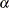for the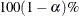confidence limits

Computes confidence limits for regression parameters

Displays covariance matrix

Specifies the denominator degrees of freedom

Displays the Hessian matrix

Displays the inverse of the Hessian matrix

Computes confidence limits for the exponentials of the regression parameters

Computes the ratio of two standard errors for the regression coefficients

Specifies tolerance for testing singularity

Specifies the method of handling ties in failure times

Computes the ratio of two variances for the regression coefficients

ALPHA=sets the level of the confidence limits for the estimated regression parameters and the hazard ratios. The value of alpha must be between 0 and 1, and the default is 0.05. A confidence level ofproduces% confidence limits. The default of ALPHA=0.05 produces 95% confidence limits.

The ALPHA= option has no effect unless the CLPARM or RISKLIMITS option is also specified.

CLPARM

produces confidence limits for regression parameters of Cox proportional hazards models. The confidence coefficient can be specified with the ALPHA= option. Classification main effects that use parameterizations other than REF, EFFECT, or GLM are ignored. See Confidence Intervals for details.

COVB

displays the estimated covariance matrix of the parameter estimates.

DF=value | keyword

specifies the denominator degrees of freedom for hypothesis tests and the degrees of freedom to use for confidence limits. If a value is specified, it must be a nonnegative number. By default, PROC SURVEYPHREG computes the degrees of freedom as described in the section Degrees of Freedom. Instead of a value, you can specify one of the following key words:

NONE

specifies the denominator degrees of freedom to be infinite. Use this option if you want to compute chi-square tests and normal confidence intervals. This option is applicable to both the Taylor series linearization and the replication methods.

computes the denominator degrees of freedom as the number of clusters (or observations if no CLUSTER statement is specified) minus the number of strata (or one if no STRATA statement is specified) minus the number of nonsingular parameters plus one in the model. This option can be useful if you are fitting a model with many parameters relative to the number of clusters minus the number of strata. See Korn and Graubard (1999, section 5.2) for further details. This option is applicable only for the Taylor series linearization method.

ALLREPS

computes the denominator degrees of freedom for replication methods by using the total number of replicate samples. By default, PROC SURVEYPHREG computes the denominator degrees of freedom based on the number of replicate samples used. See Degrees of Freedom for details.

HESS

displays the last evaluation of the Hessian matrix.

INVHESS

displays the inverse of the Hessian matrix that is evaluated at the estimated regression parameters.

RISKLIMITS
RL

produces confidence limits for hazard ratios and related quantities. See the section Hazard Ratios for details. The confidence coefficient can be specified with the ALPHA= option. Great care needs to be taken with any interpretation of the estimates and their confidence limits if interaction effects are involved in the model or if parameterizations other than REF, EFFECT, or GLM are used.

SERATIO=ALL | MODEL | IND

computes the ratio of two standard errors for the regression parameters. The standard error in the numerator uses the complete design information that you specify. You can specify the following options to compute different standard errors for the denominator:

ALL

requests both MODEL and IND standard error ratios.

MODEL

computes the standard errors in the denominator as the square root of the diagonals of the inverse Hessian matrix evaluated at the estimated regression parameters. See the section Variance Ratios and Standard Error Ratios for more information.

IND

computes the standard errors in the denominator by ignoring stratification and clustering. See the section Variance Ratios and Standard Error Ratios for more information.

SINGULAR=value

specifies the singularity criterion for determining linear dependencies in the set of explanatory variables. The default value is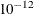.

TIES=method

specifies how to handle ties in the failure time. The available methods are as follows:

BRESLOW

uses the approximate partial likelihood of Breslow (1974). This is the default value.

EFRON

uses the approximate partial likelihood of Efron (1977).

If there are no ties, both methods result in the same likelihood and yield identical estimates. The default, TIES=BRESLOW, is the most efficient method when there are no ties.

specifies variance adjustment factors. You can use the following key words:

DF

requests degrees of freedom adjustmentin the computation of the matrixfor the Taylor series linearization variance estimation. By default, VADJUST=DF.

NONE

excludes the degrees of freedom adjustmentfrom the computation of the matrixfor the Taylor series linearization variance estimation.

AVGREPSS

use the average sum of squares from all the usable replicate samples for the unusable replicates. This option is applicable only for the jackknife replication method. AVGREPSS multiplies the default jackknife variance estimator by the factor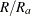, where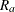is the number of usable replicates and R is the total number of replicates. See the section Variance Adjustment Factors for details.

VARRATIO=ALL | MODEL | IND

computes the ratio of two variances for the regression parameters. The variance in the numerator uses the complete design information. You can specify the following options to compute different variances for the denominator:

ALL

requests both MODEL and IND variance ratios.

MODEL

computes the variances in the denominator as the diagonals of the inverse Hessian matrix evaluated at the estimated regression parameters. See the section Variance Ratios and Standard Error Ratios for more information.

IND

computes the variances in the denominator by ignoring stratification and clustering. See the section Variance Ratios and Standard Error Ratios for more information.